Warning: recursive definition of name

This occurs when you attempt to assign a variable a value which contains the name of that variable.

> x:= 'x':
x:= x + 1;

Warning, recursive definition of name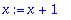Despite the warning, the assignment is performed. But an attempt to evaluate x produces an error:

> x;

Error, too many levels of recursion

To evaluate this, Maple first replaces x by its value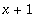. But thenmust be evaluated, obtaining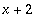. The process continues in what would be an infinite loop, except that Maple runs out of stack space and produces the error message.

The construction x:= x+1 would have been legitimate if x had originally been assigned a value (not depending on x itself). Then the x on the right side would have been evaluated first, and the result would be simply to increase the value of x by 1.

> x:= 3:
x:= x+1;Thus a common case where this warning arises is when you attempt to change the value of a loop variable, but forget to give this variable an initial value.

The warning also does not arise in defining a function. Recursive definitions of functions can be quite legitimate. Of course you should provide some way of avoiding an infinite loop in evaluating the function.

> fact:= t -> t*fact(t-1);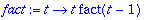> fact(0):= 1:

> fact(10);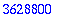Maple Advisor Database R. Israel, 1997